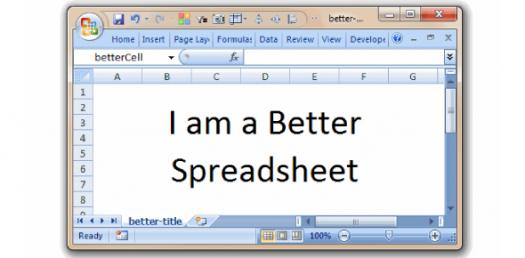# Spreadsheets Quiz: Can You Pass This Test?

Approved & Edited by ProProfs Editorial Team
At ProProfs Quizzes, our dedicated in-house team of experts takes pride in their work. With a sharp eye for detail, they meticulously review each quiz. This ensures that every quiz, taken by over 100 million users, meets our standards of accuracy, clarity, and engagement.
| Written by Tazibordercollie
T
Tazibordercollie
Community Contributor
Quizzes Created: 7 | Total Attempts: 39,802
Questions: 5 | Attempts: 333SettingsBelow is a Spreadsheets Quiz Can You Pass This Test? Most people do not know how to create a spreadsheet but before they do that it is important to know the parts of one and how you can use it. Take up the quiz below id you think you know the basics surrounding this computer application and how high you will score.

• 1.

### 1. What is a spreadsheet?

• A.

A word processing piece of software

• B.

A sheet of paper spread over a surface

• C.

A piece of software which is used to work out calculations

• D.

A database

C. A piece of software which is used to work out calculations
Explanation
A spreadsheet is a piece of software that is used to work out calculations. It allows users to organize and analyze data in a tabular format, perform mathematical calculations, create charts and graphs, and automate repetitive tasks. Spreadsheets are commonly used in various fields such as finance, accounting, and data analysis. They provide a convenient and efficient way to manage and manipulate numerical data.

Rate this question:

• 2.

### In the answer box, type the number and then the word, like so: 2. spreadsheet. A 1. _ _ _ _ _ _ _ is used to do every single calculation in the spreadsheet. A formula can be as simple as adding up two numbers in different  2. _ _ _ _ _ or it can be as complex as working out a statistical result from millions of pieces of data collected over months. The reason that formulae are so important is that once you have set them up, they will track any changes that you make in the 3. _ _ _ _ _ _ _ _ _ _ _. A spreadsheet formula must start with an 4. _ _ _ _ _ _ sign (=). It is the trigger to tell the spreadsheet that a calculation is needed.

1. formula 2. cells 3. spreadsheet 4. equals
Explanation
A formula is used to perform calculations in a spreadsheet. It can be simple, like adding two numbers in different cells, or complex, like analyzing large amounts of data. Formulae are important because they automatically update when changes are made to the spreadsheet. A spreadsheet formula always starts with an equals sign (=), which indicates that a calculation is being performed. Therefore, the correct answer is 1. formula, 2. cells, 3. spreadsheet, and 4. equals.

Rate this question:

• 3.

### Which of the following isn’t a part of a spreadsheet?

• A.

Column number

• B.

Row Number

• C.

Column Letter

• D.

A. Column number
Explanation
The column number is actually a part of a spreadsheet. In a spreadsheet, data is organized in a grid-like structure with columns and rows. Each column is identified by a unique number, allowing users to reference and manipulate specific columns of data. Therefore, the correct answer should be a component that is not a part of a spreadsheet, but the given answer of "Column number" is incorrect.

Rate this question:

• 4.

### Which of the following isn’t a part of a spreadsheet?

• A.

Decimal

• B.

Fractions

• C.

Booleans

• D.

Functions

D. Functions
Explanation
Functions are a fundamental component of spreadsheets, allowing users to perform calculations and manipulate data. They are used to automate tasks, perform mathematical operations, and analyze data. Functions in spreadsheets can range from simple mathematical calculations to complex statistical analysis. Therefore, the statement "Functions" is not a part of a spreadsheet is incorrect.

Rate this question:

• 5.

### The placement of information within a cell at the left edge, right edge, or centered is :

• A.

Alignment

• B.

Indentation

• C.

Placement

• D.

IdentificationBack to top